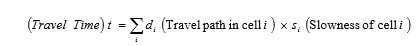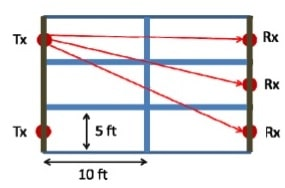# PTE-505 Inverse Modeling for Dynamic Data Integration

## Homework 2

1. [30%] The stock tank original oil-in-place (STOOIP) is approximated using the following equation:

STOOIP(stb) = 7758 Ah∮So/Bo

For a given field, A = 140 acers and Bo = 1.4 and the relation between porosity, oil saturation and depth is given as:

0.8∮2 + 0.3 So2 + 0.0098h = 0.48

1. Calculate the maximum and minimum oil in place (STOOIP) for this field given the above information.
2. If in deriving the relation between porosity, thickness, and saturation there is a 2% error in any of these variables, what would be the possible range of STOOIP?

2. [70%] In a cross-well tomography between two wells, an array of seismic sources are placed inside one well and a second array of receivers are placed in the second well. Each source generates a seismic wave that propagates through the reservoir and recorded by the receivers in the second well. For simplicity we assume there is no reflection or refractions, which means that rays travel straight lines from source to receivers. The total travel time for each ray depends on the slowness (1/velocity) property of the medium and the distance the ray travels inside that medium, per the following simple equation:i) Assuming we have only 2 sources in one well and 3 receivers in the other, write the matrix form that related the arrival times to medium slowness assuming each grid cell has a dimension 10ft × 5ft.Now consider the following problem. Two vertical wells are located 500ft apart. Ten (10) seismic sources are placed in one well (on the left) and ten (10) receivers are located in the other (on the right), resulting in 100 arrival time data. We discretize the problem into a 50 × 50 grid of 10ft × 10ft blocks resulting in 2500 model parameters. The G matrix and measured data, d, are given in file TravelTimes.mat are posted to the DEN system.

ii) Use the generalized linear least square formulation to solve the problem in MATLAB to find the slowness map. Can you identify the location and extend of oil spill?

iii) Without using any prior information, assume the slowness map is smooth and formulate the problem and derive the closed-form solution for this problem in matrix notations. Solve the problem in MATLAB to find the slowness map.